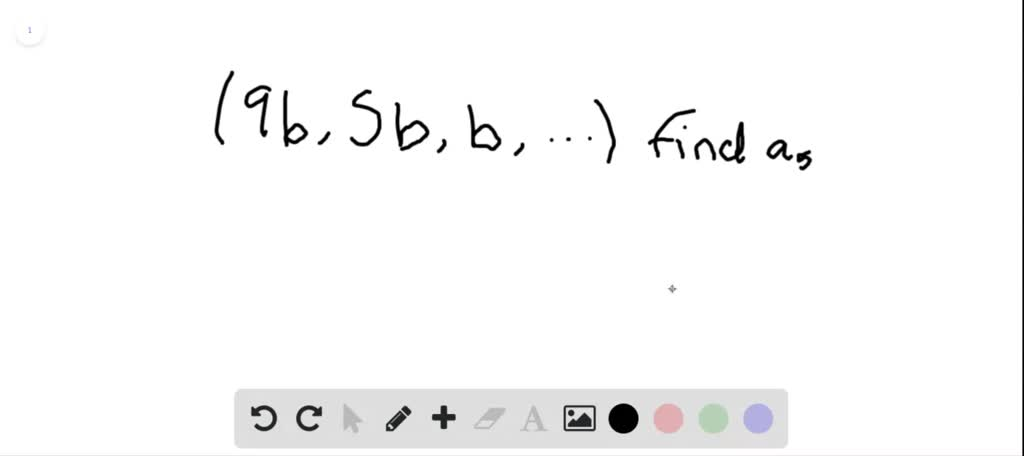1

# Find the $5^{\text { th }}$ term of the arithmetic sequence $\{9 b, 5 b, b, \ldots\}$...

## Question

###### Find the $5^{\text { th }}$ term of the arithmetic sequence $\{9 b, 5 b, b, \ldots\}$

Find the $5^{\text { th }}$ term of the arithmetic sequence $\{9 b, 5 b, b, \ldots\}$